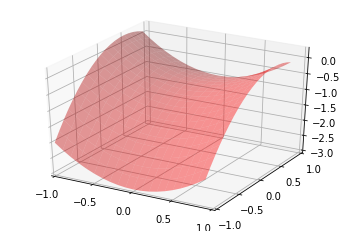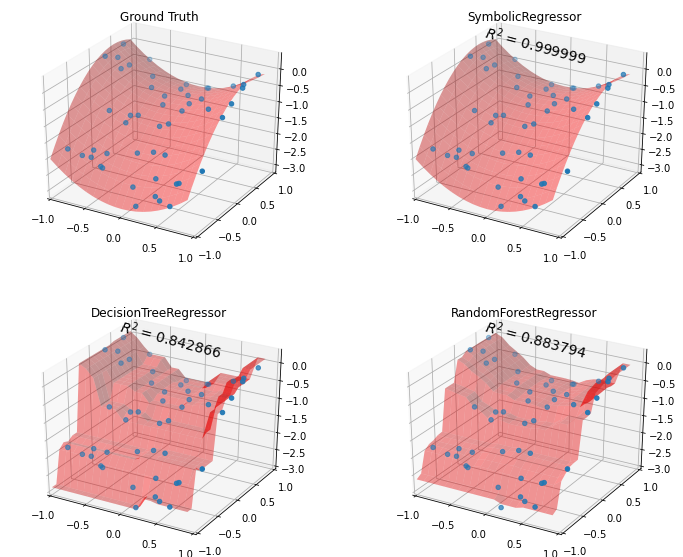# Python: Symbolic Regression¶

## A Basic Example¶

### Overview¶

Scientific progress, especially in the physical sciences, is a story of hypothesis producing testable predictions that are then either confirmed or rejected by observations (i.e. data). Even in predictive modeling, we generally fit a given model to observed data. What if we could go the other way?

What if we could take the data, and find the equation that would most closely have produced the data that we observe? Symbolic regression offers us an opportunity to do just that. It searches the solution spaces of possible equations, by combining mathematical operators with functional forms in a somewhat random manner guided by evolutionary success (e.g. piecing together the most promising mathematical forms using genetic algorithms). In this way, the resulting equation is free from assumptions (e.g. assuming the model is linear, a-la linear regression), or biases about how the dependent variable is related to the independent variables, etc.

The following example is recreated from gplearn's library, available here: https://gplearn.readthedocs.io/en/stable/examples.html#symbolic-regressor

### Data Exploration¶

We generate some data using an assumed equation and then plot it in 3D.

In :
#loading packages & dependencies
#When the %pylab magic function is entered at the IPython prompt, it triggers the import of various modules within Matplotlib.
%pylab inline
from gplearn.genetic import SymbolicRegressor
from sklearn.ensemble import RandomForestRegressor
from sklearn.tree import DecisionTreeRegressor
from sklearn.utils.random import check_random_state
from mpl_toolkits.mplot3d import Axes3D
import matplotlib.pyplot as plt
import numpy as np
import graphviz

Populating the interactive namespace from numpy and matplotlib


We generate some data using the following equation: $$y = X_0^2-X_1^2+X_1-1 \\$$ The goal will be to then use the gplearn package and see if we can recover the equation from the data that it generated.

In :
# Ground truth
x0 = np.arange(-1, 1, .1)
x1 = np.arange(-1, 1, .1)
x0, x1 = np.meshgrid(x0, x1)
y_truth = x0**2 - x1**2 + x1 - 1 #true function

ax = plt.figure().gca(projection='3d')
ax.set_xlim(-1, 1)
ax.set_ylim(-1, 1)
ax.set_xticks(np.arange(-1, 1.01, .5))
ax.set_yticks(np.arange(-1, 1.01, .5))
surf = ax.plot_surface(x0, x1, y_truth, rstride=1, cstride=1, color='red', alpha=0.4)
plt.show()In :
rng = check_random_state(0)

# Training samples
X_train = rng.uniform(-1, 1, 100).reshape(50, 2)
y_train = X_train[:, 0]**2 - X_train[:, 1]**2 + X_train[:, 1] - 1

# Testing samples
X_test = rng.uniform(-1, 1, 100).reshape(50, 2)
y_test = X_test[:, 0]**2 - X_test[:, 1]**2 + X_test[:, 1] - 1


### Symbolic Regression¶

We apply gplearn's symbolic regression function below:

In :
est_gp = SymbolicRegressor(population_size=5000, #the number of programs in each generation
generations=30, stopping_criteria=0.01, #The required metric value required in order to stop evolution early.
p_crossover=0.7, p_subtree_mutation=0.1,
p_hoist_mutation=0.05, #0.05, The probability of performing hoist mutation on a tournament winner. Hoist mutation takes the winner of a tournament and selects a random subtree from it. A random subtree of that subtree is then selected and this is ‘hoisted’ into the original subtrees location to form an offspring in the next generation. This method helps to control bloat.
p_point_mutation=0.1,
max_samples=0.9, verbose=1,
parsimony_coefficient=0.01, random_state=0)
est_gp.fit(X_train, y_train)

    |   Population Average    |             Best Individual              |
---- ------------------------- ------------------------------------------ ----------
Gen   Length          Fitness   Length          Fitness      OOB Fitness  Time Left
0    38.13          458.578        5         0.320666         0.556764      2.75m
1     9.97          1.70233        5         0.320202         0.624787      1.44m
2     7.72          1.94456       11         0.239537         0.533148      1.39m
3     5.41         0.990157        7         0.235676         0.719906      1.48m
4     4.66         0.894443       11         0.103946         0.103946      1.24m
5     5.41         0.940242       11         0.060802         0.060802      1.21m
6     6.78          1.09536       11      0.000781474      0.000781474      1.13m

Out:
SymbolicRegressor(const_range=(-1.0, 1.0), feature_names=None,
init_depth=(2, 6), init_method='half and half',
low_memory=False, max_samples=0.9,
metric='mean absolute error', n_jobs=1, p_crossover=0.7,
p_hoist_mutation=0.05, p_point_mutation=0.1,
p_point_replace=0.05, p_subtree_mutation=0.1,
parsimony_coefficient=0.01, population_size=5000,
random_state=0, stopping_criteria=0.01, tournament_size=20,
verbose=1, warm_start=False)
In :
print(est_gp._program)

sub(add(-0.999, X1), mul(sub(X1, X0), add(X0, X1)))


#### Making Sense of the output¶

The output (above) is in a strange sort of syntax, so let's parse it. The 'sub', 'add', and 'mul' mean subtraction, addition and multiplication respectively. Here is how we can simply the above expression using some algebra: $$y = (-0.999+X_1) - ((X_1-X_0)\times(X_0+X_1)) \\ y = X_1-0.999-(X_1X_0+X_1^2-X_0^2-X_0X_1) \\ y = X_0^2-X_1^2+X_1-0.999$$

That's amazing! We recovered almost exactly (the only difference being 0.999 instead of 1.0 - I think we can ignore that!) the equation that we assumed in order to generate the data.

In the step below, we see how a couple of other methods (e.g. decision tree regressor and random forests) perform on the data as far as predictions go:

In :
est_tree = DecisionTreeRegressor()
est_tree.fit(X_train, y_train)
est_rf = RandomForestRegressor(n_estimators=10)
est_rf.fit(X_train, y_train)

Out:
RandomForestRegressor(bootstrap=True, ccp_alpha=0.0, criterion='mse',
max_depth=None, max_features='auto', max_leaf_nodes=None,
max_samples=None, min_impurity_decrease=0.0,
min_impurity_split=None, min_samples_leaf=1,
min_samples_split=2, min_weight_fraction_leaf=0.0,
n_estimators=10, n_jobs=None, oob_score=False,
random_state=None, verbose=0, warm_start=False)
In :
y_gp = est_gp.predict(np.c_[x0.ravel(), x1.ravel()]).reshape(x0.shape)
score_gp = est_gp.score(X_test, y_test)
y_tree = est_tree.predict(np.c_[x0.ravel(), x1.ravel()]).reshape(x0.shape)
score_tree = est_tree.score(X_test, y_test)
y_rf = est_rf.predict(np.c_[x0.ravel(), x1.ravel()]).reshape(x0.shape)
score_rf = est_rf.score(X_test, y_test)

fig = plt.figure(figsize=(12, 10))

for i, (y, score, title) in enumerate([(y_truth, None, "Ground Truth"),
(y_gp, score_gp, "SymbolicRegressor"),
(y_tree, score_tree, "DecisionTreeRegressor"),
(y_rf, score_rf, "RandomForestRegressor")]):

ax = fig.add_subplot(2, 2, i+1, projection='3d')
ax.set_xlim(-1, 1)
ax.set_ylim(-1, 1)
ax.set_xticks(np.arange(-1, 1.01, .5))
ax.set_yticks(np.arange(-1, 1.01, .5))
surf = ax.plot_surface(x0, x1, y, rstride=1, cstride=1, color='red', alpha=0.4)
points = ax.scatter(X_train[:, 0], X_train[:, 1], y_train)
if score is not None:
score = ax.text(-.7, 0.1, .1, "$R^2 =\/ %.6f$" % score, 'x', fontsize=14)
plt.title(title)

plt.show()### Feedback¶

If you have ideas on how to improve this post, please let me know: https://predictivemodeler.com/feedback/